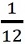# Comparing a Numerical Expression With a Number

A numerical expression is a mathematical statement involving only numbers and one or more arithmetic operation symbols(+, −, ×, ÷).

A numerical expression always represents a particular number.

For example, the numerical expression 5 + 20 − 9 is equal to the number 16 when simplified.

Examples

The following are some examples of numerical expressions.

5 + 20 − 7, (4 + 3) − 7, (6 × 4) ÷ 20, 5 ÷ (30 × 3), 9 × (41 + 4)

Use <, >, or = to compare the numerical expression and the number.

 5 ÷ (20 × 3) 8

### Solution

Step 1:

5 ÷ (20 × 3) = 5 ÷ 60 =Step 2:< 8

Step 3:

So 5 ÷ (20 × 3) < 8

Use <, >, or = to compare the numerical expression and the number.

 5 x (42 + 3) 90

### Solution

Step 1:

5 × (42 + 3) = 5 × 45 = 225

Step 2:

225 > 90

Step 3:

So 5 × (42 + 3) > 90

Use <, >, or = to compare the numerical expression and the number.

 (2 + 3) - 7 6

### Solution

Step 1:

(2 + 3) − 7󠄀 = 5 − 7 = − 2

Step 2:

− 2 < 6

Step 3:

So (2 + 3) − 7󠄀< 6

Use <, >, or = to compare the numerical expression and the number.

 4 + 20 - 7 14

### Solution

Step 1:

4 + 20 − 7󠄀 = 17

Step 2:

17 > 14

Step 3:

So 4 + 20 − 7󠄀 > 14